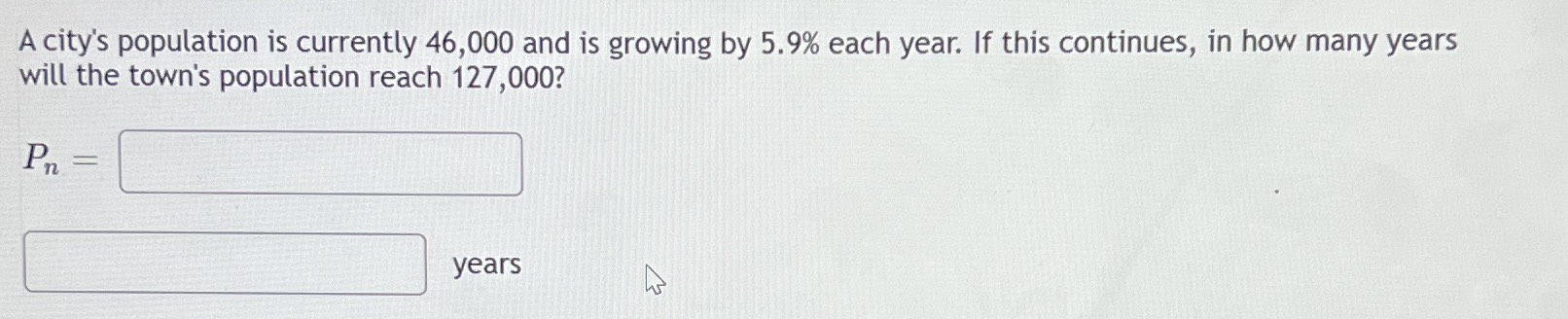### ¿Todavía tienes preguntas de matemáticas?

Pregunte a nuestros tutores expertos
Algebra
PreguntaA city's population is currently $$46,000$$ and is growing by $$5.9 \%$$ each year. If this continues, in how many years will the town's population reach $$127,000$$ ?

$$P _ { n } =$$   years

$$P_n= 46000(1.059)^n$$
$$n= 17.72 \text{ years}$$